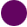SEARCH HOMEMath Central Quandaries & QueriesQuestion from Laura, a parent: On a math test Grace needs to look at an array and write a division sentence for the array. Grace writes 18/3=6 on her answer sheet. Describe what the array on Graces test looks like.Hi Laura,

I'm going to change the problem slightly.

On a math test Larry needs to look at an array and write a division sentence for the array. Larry writes 15/5 = 3 on his answer sheet. Describe what the array on Larry test looks like.

I would rewrite Larry's division sentence as a multiplication sentence. That is I would rewrite 15/5 = 3 as 5 × 3 = 15 or perhaps 3 × 5 = 15. I can draw arrays for these sentences,andThe first is a 5 by 3 array and the second is a 3 by 5 array. (Mathematicians always write the number of rows first and the number of columns second.) Thus my response to the question about Larry's array would be to say it is either a 5 by 3 array or a 3 by 5 array.

I hope this helps,
PennyMath Central is supported by the University of Regina and The Pacific Institute for the Mathematical Sciences.